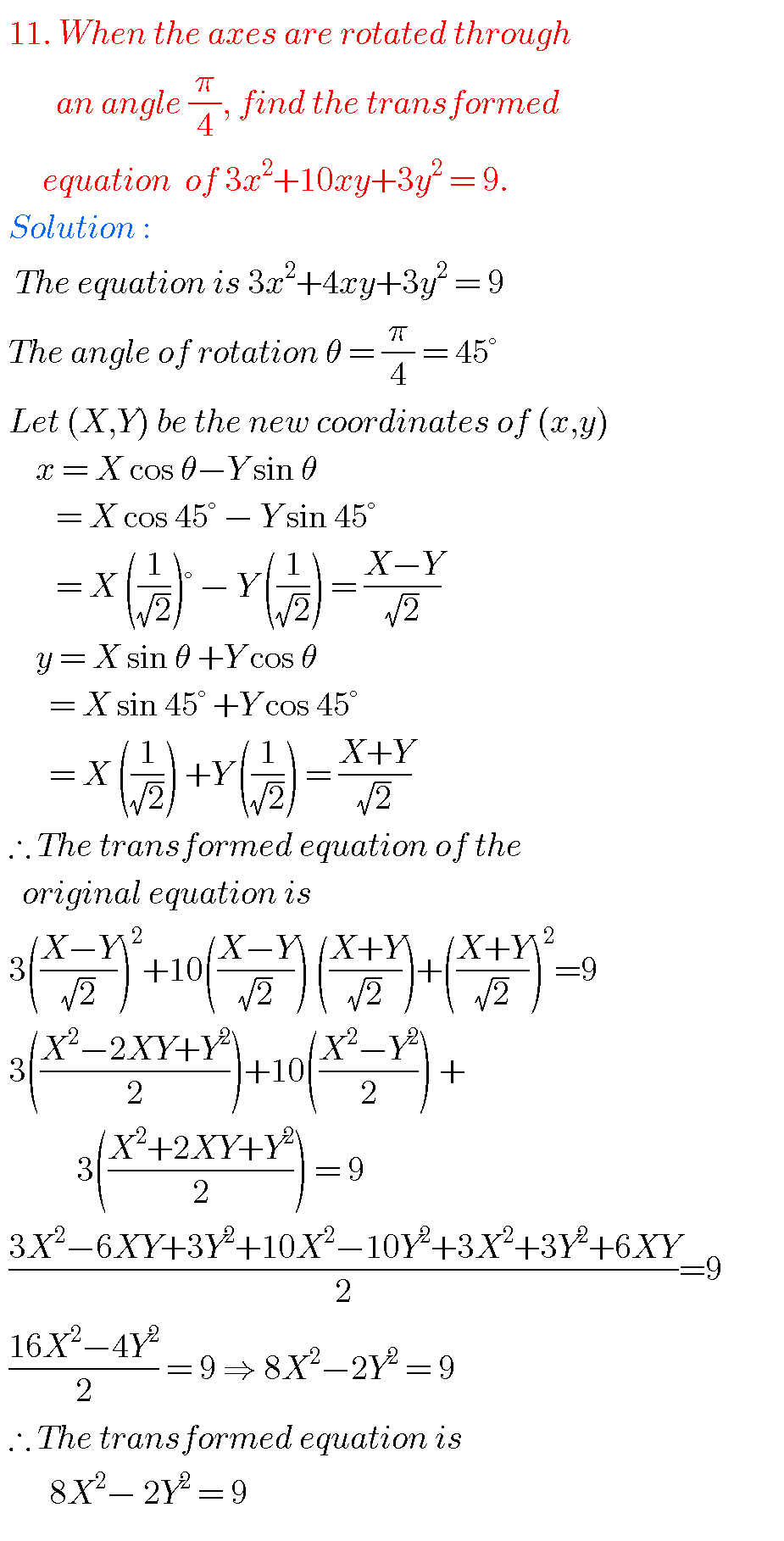## Transformation of Axes,Intermediate first year 1B chapter 2 problems with solutions

TRANSFORMATION OF AXES Inter, INTERMEDIATE FIRST YEAR 1B SOLUTIONS Mathematics Intermediate1A Transformation of axes solutions for examination purpose are given below. These are very simple to understand. Study the textbook lesson Transformation of axes very well. Observe the example problems and solutions given in the textbook. Transformation of axes textbook solutions Exercise 2(a) You can …